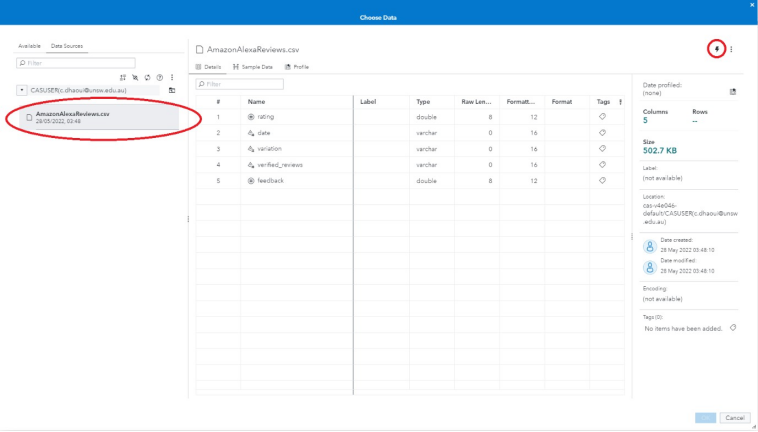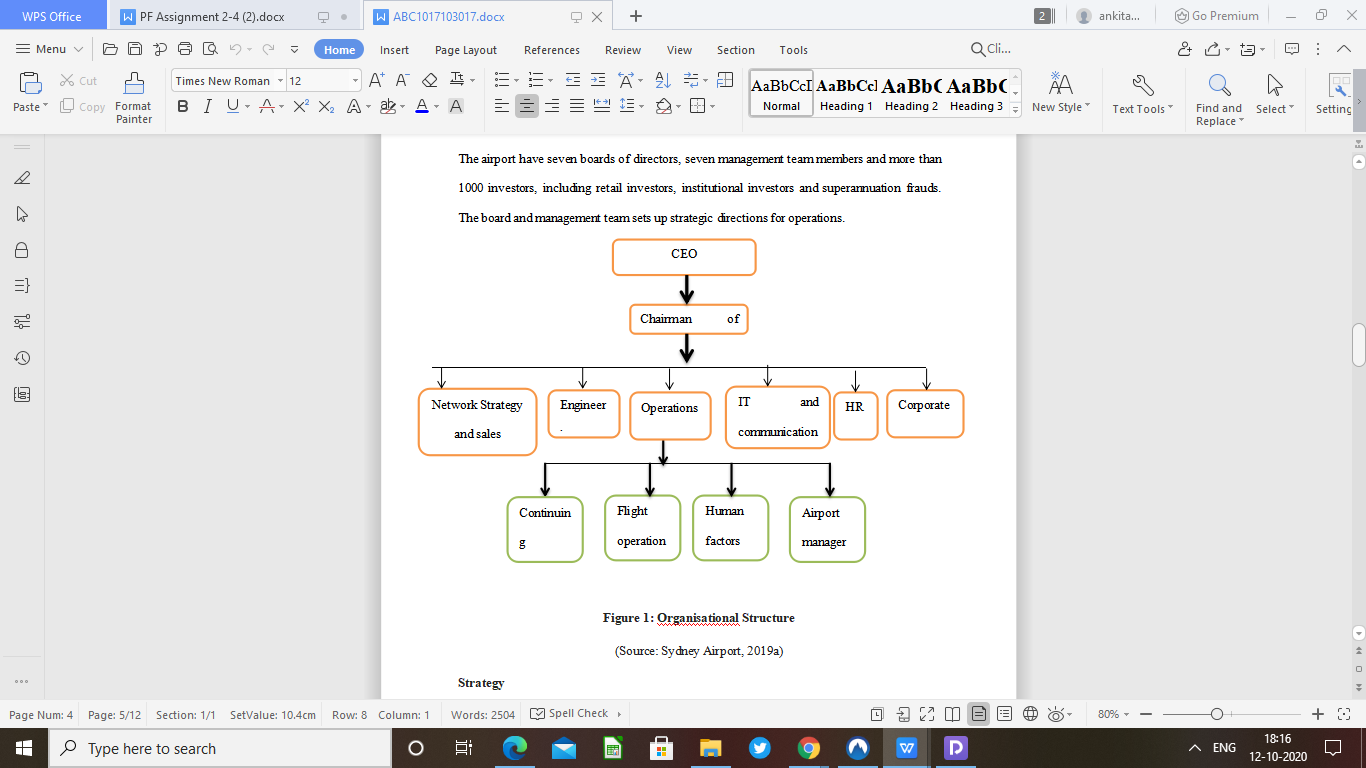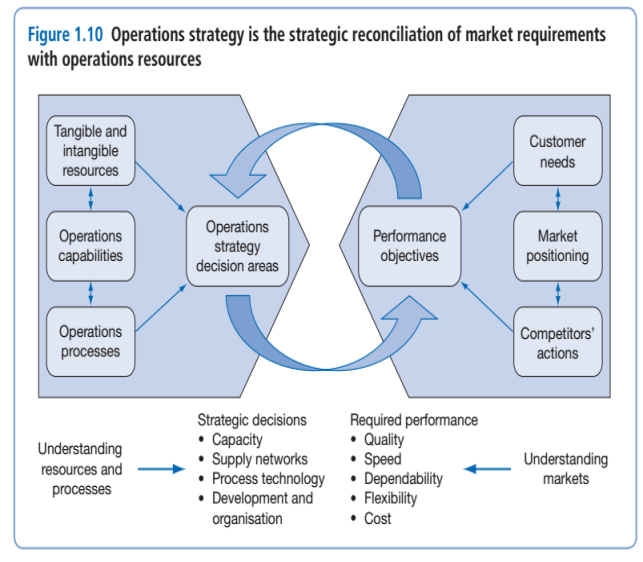# Statistics For Life And Social SciencePages: 4Words: 890

## Question :

MATH1041 Computing assignment

"BW" "HTL" "SEX"
50.58 0.88 1
52.45 1.38 1
45.21 1.1 1
63.9 1.83 1
58.22 1.38 1
51.05 1.03 1
60.88 1.57 0
54.46 1.23 0
58.31 0.99 0
49.7 1.23 0
54.27 1.27 1
54.09 1.37 0
48.27 1.58 1
58.57 1.63 1
57.23 0.58 0
58.86 0.98 1
60.17 1.55 1
50.24 2.93 1
50.91 0.95 0
50.37 1.09 0
48.31 1.07 1
61.16 1.83 0
44.52 1.54 1
47.58 1.24 0
59.15 0.91 1
60.12 1.71 1
54.72 1.54 0

Format

Here are some more details that may assist you:

• Regarding the overall assignment structure, this is up to you, just remember to keep it clear and concise. If you are answering questions in the given order (that is, 1a), b), etc.), then this is fine. You don’t need to re-write the assignment question again.

• You are required to type up your entire assignment (rather than scanning and taking screenshots). If you are using Word you should use the equation editor for any maths notation. If you don’t have Word then please use the School computers. Please convert and submit your assignment in pdf.

• You are asked to produce SIX graphs/plots for this assignment. You are required to produce these in RStudio. You may want to use the par(mfrow=c(2,3)) function to construct all six graphs per plot (this is optional), see Section R1.4 “Transforming data using RStudio”of the RStudio “How-To-Manual” available on Moodle.

• We recommend adding some working out for some of the questions involving calculations. But try to keep your solutions brief and concise (since there is a page limit). It’s good practice for the exam and in case you get the wrong answer you have some workings to gain marks from. Your working could consist of RStudio commands or perhaps the main steps on how you arrived at your answer. You don’t need to add all of your R-code! • Keeping your results to 2 or 3 decimal places should be fine.

• There is no requirement for font size and line spacing but obviously don’t make things too small.

Scenario

A team of researchers were interested in studying the impacts of drought on sheep livestock in farms around New South Wales and Queensland, Australia. In particular, the researchers wanted to compare the average body weight of sheep from five years ago (when there was little drought) to now (Spring, 2018) where drought is of serious concern. To obtain their data, the research team decided to collect a random sample of sheep from a very large sheep population on a farm affected by the drought. This random sample of data consists of sheep body weight measurements (measured in kilograms), head-to-tail length measurements (measured in metres) and their gender (male/female). The text file contains your unique data of length n in separate rows consisting of 3 variables: BW which corresponds to sheep body weights, HTL which corresponds to sheep head-to-tail lengths, and SEX which corresponds to gender (0 = Female and 1 = Male). Your job is to assist the research team by analysing the data set provided to you.

The questions you need to answer in your assignment submission are given below. Please make sure your assignment is converted to pdf format.

1. (a) Calculate the sample mean and sample standard deviation of your sample of sheep body weight (BW) measurements.

(b) Produce a normal quantile plot of your sample of sheep body weight measurements (see Section R2.6 “How to produce a normal quantile plot using RStudio”). Include this plot in your submitted assignment, properly labelled.

(c) By referring to the normal quantile plot obtained in Part 1b briefly discuss if the sheep body weights are approximately normally distribution.

2. Let µ be the population mean body weight (in kg) of sheep (of any gender) on the farm now (Spring, 2018). The research team decided to compare the current sheep mean body weight with the mean from five years ago. The known mean body weight for sheep from five years ago was 60kg.

(a) Test the hypothesis that µ is equal to 60. You must summarize all steps: state the null (H0) and alternative hypotheses (Ha) relevant to the research objectives stated in this scenario, the value of a suitable test statistic, the sampling distribution for this statistic, a P-value, your summary of significance and conclusion in plain language.

(b) Some assumptions need to be made for the sampling distribution of the test statistic (as given in Part 2a) to be valid. State these assumptions.

(c) Discuss whether the assumptions from Part 2b are satisfied?

(d) Produce a 95% confidence interval for µ, the mean body weight of sheep. For this question you may assume that it is appropriate to use a t-distribution. Make sure you write down all the required steps to calculate this interval. Does this confidence interval include the value 60? Explain whether your confidence interval is consistent with your conclusions from the hypothesis test in Part 2a.

3. The research team were also interested in studying:

• the relationship between body weight and gender; and

• the relationship between body weight and head-to-tail length.

(a) Produce a comparative boxplot for sheep body weight against gender. Include this plot in your submitted assignment, properly labelled.

(b) Describe any differences or similarities in the distribution of body weight of sheep for the different genders using your comparative boxplot from Parts 3a.

(c) Construct an appropriate graphical summary to visualize the relationship between body weight and head-to-tail length. Include this plot in your assignment, properly labelled.

(d) Summarize the key features of your plot from Part 3c.

(e) Suggest an appropriate numerical summary to quantify the linear relationship between body weight and head-to-tail length. Report and comment on this value.

(f) The research team wanted to predict sheep body weight from head-to-tail length measurement by fitting a linear regression model. Would you recommend the research team do this? Explain briefly.

4. The research team decided to investigate the head-to-tail length (HTL) measurement in more detail.

(a) Produce a five number summary for the HTL measurements.

(b) Produce a histogram for the HTL measurements. Include this histogram in your submitted assignment properly labelled.

(c) In MATH1041, we looked at the effect of transforming data. Using the HTL measurements, perform:

(1) a log transformation; and

(2) a square-root transformation, and produce a histogram for each of these. Include these histograms in your submitted assignment properly labelled.

(d) Summarize the key features of each histogram from Parts 4b and 4c (that is, the raw data, and each of the transformations). Please comment on central location, spread, and (any) skewness/symmetry.

(e) Do you think these transformations reduced any skewness? Explain briefly.

1 a) The sample mean and standard deviation of the sheep body weight in r studio is calculated as follows:

file = "data.csv"

mean(data\$BW)

sd(data\$BW)1 b) The normal quantile graph of the sheep body weight in r is as follows:

# Normal  Quantile graph

qqnorm(data\$BW, main='Normal Q-Q Plot')

qqline(data\$BW)1 c) The above diagram shows us that the point in the graph is not present on the straight line while we know that the graph to be normal , point should be nearer or almost on the straight line. Here this is not the case. So we can say that it is not normal distribution.
2)

a) In this problem the population mean body weight of sheep is calculated above and we get the value using mean(data\$BW). The value we get is 54.1963 while the mean value is 60 age  five years. Now to check the hypothesis is it is equal to 60. We have used the t-test. But the result we get is different. The assumed hypothesis is as follows:

H0: mu =60

H1: mu != 60

The r script for the t test is as follows:

t.test(data\$BW, mu=60)b) The t-test analysis is used to compare the two mean value  while we know that T-distribution is basically a continuous distribution which arises from the estimation of the mean. The assumptions which is considered regarding

c) As we have observed that we are not getting the points which mentioned above in the question is not satisfying accordingly, because our sample size is very less. The second point is when we have drawn the graph we are not getting the bell shaped curve and our data is not continuous. So we can clearly say that assumptions made by us is not satisfying.

d)  To produce the 95% percent confidence interval, the mean body weight of sheep. In this we have used the t-test for the body weight of sheep. The R-script of the mean body weight of sheep is as follows:

file = "data.csv"

t.test(data\$BW)

the result for this R script is:In this we have observed that it has not included the confidence interval value 60. In this confidence interval we have observed one thing is that the mean which we got is 52.08 to 56.30 while the actual mean of the sheep body weight is 54.19, which is between the confidence interval mean, while the mean is not equal to 60. So we can say that the alternative hypothesis which we have considered is true, and our data is consistent then we are getting our mean between the confidence interval.

3) a) The comparative boxplot for sheep body against the gender using r is as follows:

file = "data.csv"

boxplot(split(data\$BW, data\$SEX), xlab="gender", ylab="body Weight")b) After observing the above graph, we are not getting any kind of similarities on the distribution. Because in the above graph there is no female who is below the weight 45 while in the men section we can easily say that there is male in the given distribution who is below the 45 and above 65. While in case of the women there is no woman whose body weight is greater than 65. Hence this is observation which supports my view that there is no similarities.

c) The visualization of the relationship between the body weight and head to tail length. The r script and its graph is as follows:

library(ggplot2)

file = "data.csv"

qplot(data1\$BW, data1\$HTL, data=data1, stat="summary", fun.y="sum")d)   The key features from the part 3c is there is only one point which is outside the all the point near to the y -axis. We can say it outlier. In this rest all the points is below the 2.0. this show that there is dense part which is used to describe the data. The descriptive summary statistics of the plot is calculated using the summary function. This gives the result as follows:

BW             HTL

Min.   :44.52   Min.   :0.580

1st Qu.:50.30   1st Qu.:1.050

Median :54.27   Median :1.270

Mean   :54.20   Mean   :1.348

3rd Qu.:58.72   3rd Qu.:1.560

Max.   :63.90   Max.   :2.930

e) The numerical summary to quantify the linear relationship between body weight and head-to-tail length is that we will implement the linear regression to get this. The another method to get the numerical summary is to get the mean, median and all the statistical value of the data. Which we will get using the summary function on the data.f) The implementation of the linear regression model on the attributes body weight and head-to-tail is as follows:

Regression <- lm(data\$BW ~ data\$HTL)

summary(Regression)

newdata<- data.frame(x=data\$BW)

predicted_val<-predict(Regression, newdata, type="response")

predicted_val4 a) The five number summary is a set of 5 descriptive statistics for summarizing the continuous univariate dataset. it consists the minimum, 1st quartile, median, 3rd quartile and maximum. The fivenumber summary of HTL is as follows:

fivenum(data\$HTL)b) The histogram of the HTL is as follows:hist(data\$HTL)

c) The log transformation of Head-to-Tail is as follows:

log10(data\$HTL)Square root transformation of HTL is as follows:

T_sqrt<- sqrt(data\$HTL)

T_sqrt

hist(T_sqrt)d) The central tendency of the log transformation of the histogram on the htl is coming between the 0.0 to 0.2 while the frequency is between 4 to 6 i.e. 5 and there is no more skewness part. While  the central tendency for thee square root transformation is between the 1. 2 to 1.4 while the frequency of this transformation is between 6 to8 that is 7.5.

e) According to me there is no more role of the skewness in this part. Because we can see easily the histograms and we can say that there is no role of the skewness in the dataset and its analysis part. While we know that transformation is used to reduce the skewness when we take the log transformation it reduces the right skewness due to the square transformation we reduce the left skewness. Here our dataset is small so no more role of the skewness.

Tags: